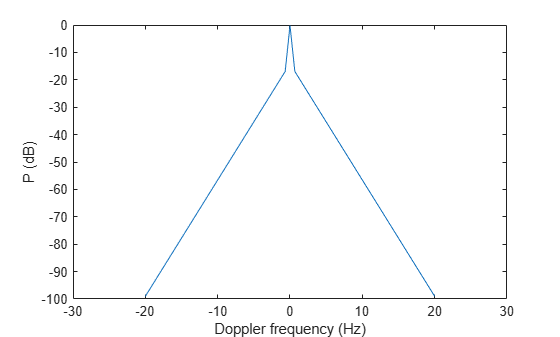# billingsleyicm

Billingsley’s intrinsic clutter motion (ICM) model

## Syntax

```P = billingsleyicm(fd,fc,wspeed) P = billingsleyicm(fd,fc,wspeed,c) ```

## Description

`P = billingsleyicm(fd,fc,wspeed)` calculates the clutter Doppler spectrum shape, `P`, due to intrinsic clutter motion (ICM) at Doppler frequencies specified in `fd`. ICM arises when wind blows on vegetation or other clutter sources. This function uses Billingsley’s model in the calculation. `fc` is the operating frequency of the system. `wspeed` is the wind speed.

`P = billingsleyicm(fd,fc,wspeed,c)` specifies the propagation speed `c` in meters per second.

## Input Arguments

 `fd` Doppler frequencies in hertz. This value can be a scalar or a vector. `fc` Operating frequency of the system in hertz. `wspeed` Wind speed in meters per second. `c` Propagation speed in meters per second. Default: Speed of light

## Output Arguments

 `P` Shape of the clutter Doppler spectrum due to intrinsic clutter motion. The vector size of `P` is the same as that of `fd`.

## Examples

collapse all

Calculate and plot the Doppler spectrum shape predicted by the Billingsley ICM model. Assume the PRF is 2 kHz, the operating frequency is 1 GHz, and the wind speed is 5 m/s.

```v = -3:0.1:3; fc = 1e9; wspeed = 5; c = physconst('LightSpeed'); fd = 2*v/(c/fc); p = billingsleyicm(fd,fc,wspeed); plot(fd,pow2db(p)) xlabel('Doppler frequency (Hz)') ylabel('P (dB)')```## References

 Billingsley, J. Low Angle Radar Clutter. Norwich, NY: William Andrew Publishing, 2002.

 Long, Maurice W. Radar Reflectivity of Land and Sea, 3rd Ed. Boston: Artech House, 2001.

## Extended Capabilities

Introduced in R2021a